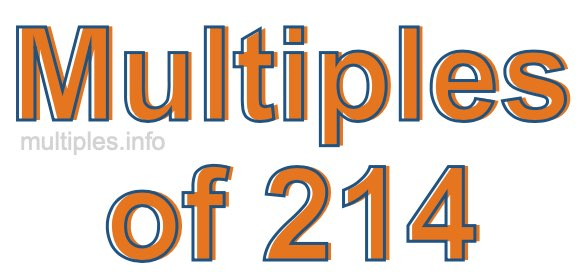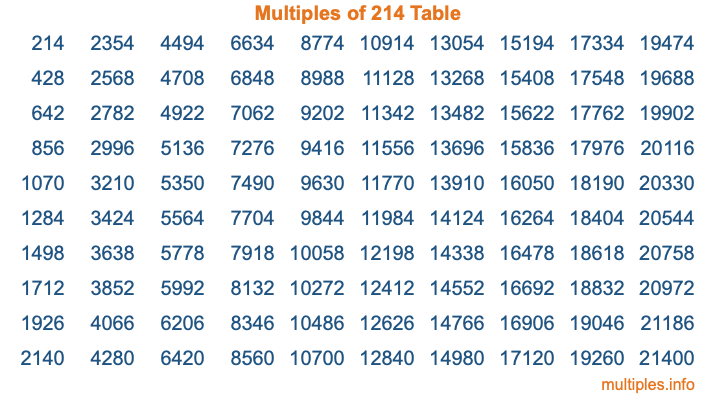Multiples of 214Welcome to the Multiples of 214 page. Here we will first teach you everything you will ever need to know about the multiples of 214, and then give you a study guide summary of everything we taught you to make sure you remember it all. Use this page to look up facts and learn information about the multiples of 214. This page will make you a multiples of two hundred fourteen expert!

Definition of Multiples of 214
Multiples of 214 are all the numbers that when divided by 214 equal an integer. Each of the multiples of 214 are called a multiple. A multiple of 214 is created by multiplying 214 by an integer.

Therefore, to create a list of multiples of 214, you start with 1 multiplied by 214, then 2 multiplied by 214, then 3 multiplied by 214, and so on for as long as you want. Thus, the list of the first five multiples of 214 is 214, 428, 642, 856, and 1070. To see a larger list of multiples of 214, see the printable image of Multiples of 214 further down on this page. We also have a category where you can choose any nth multiple of 214.

Multiples of 214 Checker
The Multiples of 214 Checker below checks to see if any number of your choice is a multiple of 214. In other words, it checks to see if there is any number (integer) that when multiplied by 214 will equal your number. To do that, we divide your number by 214. If the the quotient is an integer, then your number is a multiple of 214.

Is  a multiple of 214?

Least Common Multiple of 214 and ...
A Least Common Multiple (LCM) is the lowest multiple that two or more numbers have in common. This is also called the smallest common multiple or lowest common multiple and is useful to know when you are adding our subtracting fractions. Enter one or more numbers below (214 is already entered) to find the LCM.

Check out our LCM Calculator if you need more details about the Least Common Multiple or if you need the LCM for different numbers for adding and subtraction fractions.

nth Multiple of 214
As we stated above, 214 is the first multiple of 214, 428 is the second multiple of 214, 642 is the third multiple of 214, and so on. Enter a number below to find the nth multiple of 214.

th multiple of 214

Multiples of 214 vs Factors of 214
214 is a multiple of 214 and a factor of 214, but that is where the similarities end. All postive multiples of 214 are 214 or greater than 214. All positive factors of 214 are 214 or less than 214.

Below is the beginning list of multiples of 214 and the factors of 214 so you can compare:

Multiples of 214: 214, 428, 642, 856, 1070, etc.

Factors of 214: 1, 2, 107, 214

As you can see, the multiples of 214 are all the numbers that you can divide by 214 to get a whole number. The factors of 214, on the other hand, are all the whole numbers that you can multiply by another whole number to get 214.

It's also interesting to note that if a number (x) is a factor of 214, then 214 will also be a multiple of that number (x).

Multiples of 214 vs Divisors of 214
The divisors of 214 are all the integers that 214 can be divided by evenly. Below is a list of the divisors of 214.

Divisors of 214: 1, 2, 107, 214

The interesting thing to note here is that if you take any multiple of 214 and divide it by a divisor of 214, you will see that the quotient is an integer.

Multiples of 214 Table
Below is an image of the first 100 multiples of 214 in a table. The table is in chronological order, column by column. The first column has the first ten multiples of 214, the second column has the next ten multiples of 214, and so on.The Multiples of 214 Table is also referred to as the 214 Times Table or Times Table of 214. You are welcome to print out our table for your studies.

Negative Multiples of 214
Although not often discussed or needed in math, it is worth mentioning that you can make a list of negative multiples of 214 by multiplying 214 by -1, then by -2, then by -3, and so on, to get the following list of negative multiples of 214:

-214, -428, -642, -856, -1070, etc.

Multiples of 214 Summary
Below is a summary of important Multiples of 214 facts that we have discussed on this page. To retain the knowledge on this page, we recommend that you read through the summary and explain to yourself or a study partner why they hold true.

There are an infinite number of multiples of 214.

A multiple of 214 divided by 214 will equal a whole number.

214 divided by a factor of 214 equals a divisor of 214.

The nth multiple of 214 is n times 214.

The largest factor of 214 is equal to the first positive multiple of 214.

214 is a multiple of every factor of 214.

214 is a multiple of 214.

A multiple of 214 divided by a divisor of 214 equals an integer.

214 divided by a divisor of 214 equals a factor of 214.

Any integer times 214 will equal a multiple of 214.

Multiples of a Number
Here you can get the multiples of another number, all with the same attention to detail as we did for multiples of 214 on this page.

Multiples of
Multiples of 215
Did you find our page about multiples of two hundred fourteen educational? Do you want more knowledge? Check out the multiples of the next number on our list!